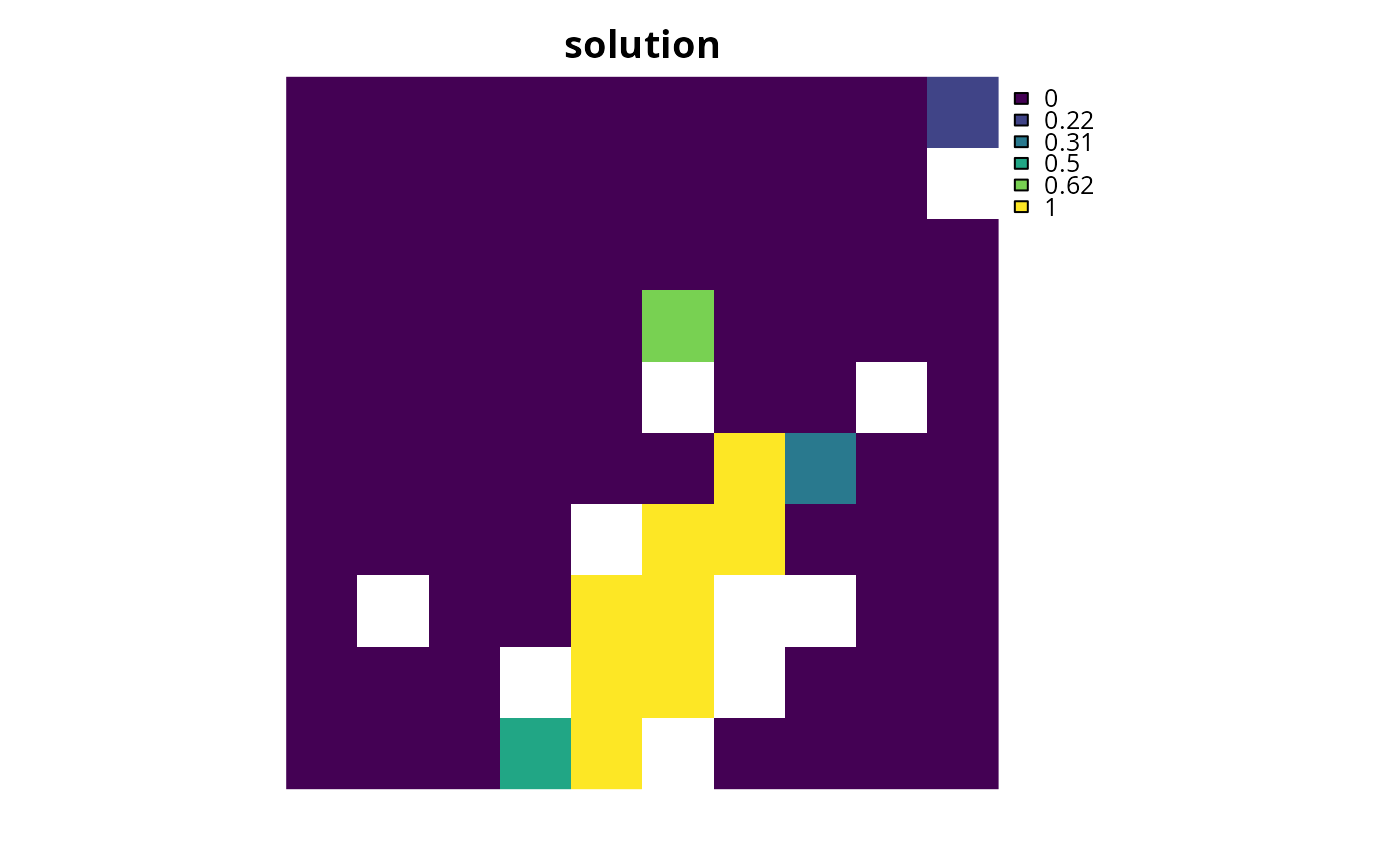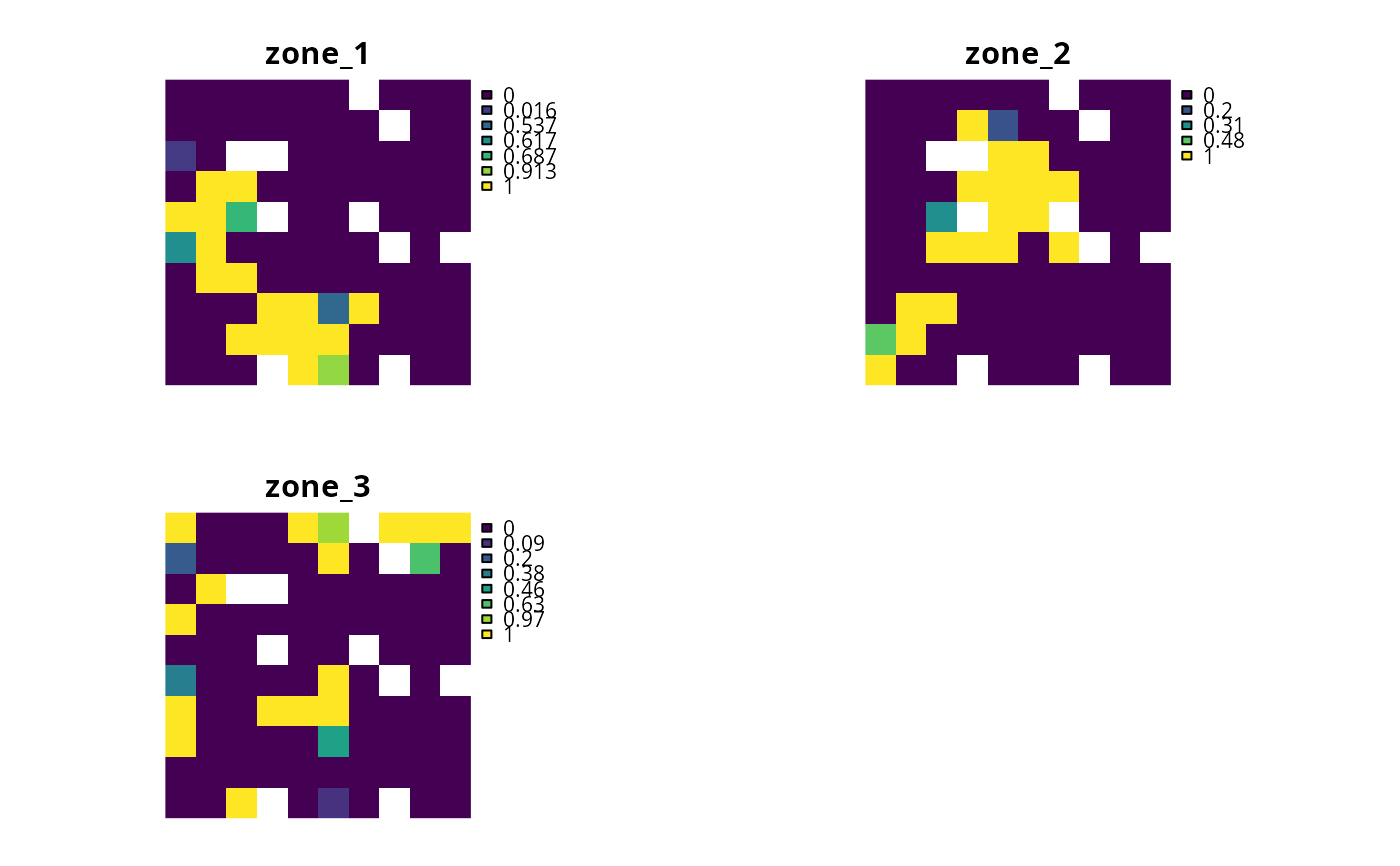Add a proportion decision to a conservation planning problem. This is a relaxed decision where a part of a planning unit can be prioritized, as opposed to the entire planning unit. Typically, this decision has the assumed action of buying a fraction of a planning unit to include in decisions will solve much faster than problems that use binary-type decisions.

## Usage

add_proportion_decisions(x)

## Arguments

x

problem() object.

## Value

An updated problem() object with the decisions added to it.

## Details

Conservation planning problems involve making decisions on planning units. These decisions are then associated with actions (e.g., turning a planning unit into a protected area). Only a single decision should be added to a problem() object. Note that if multiple decisions are added to an object, then the last one to be added will be used.

See decisions for an overview of all functions for adding decisions.

Other decisions: add_binary_decisions(), add_semicontinuous_decisions()

## Examples

# \dontrun{
# set seed for reproducibility
set.seed(500)

sim_pu_raster <- get_sim_pu_raster()
sim_features <- get_sim_features()
sim_zones_pu_raster <- get_sim_zones_pu_raster()
sim_zones_features <- get_sim_zones_features()

# create minimal problem with proportion decisions
p1 <-
problem(sim_pu_raster, sim_features) %>%

# solve problem
s1 <- solve(p1)

# plot solutions
plot(s1, main = "solution", axes = FALSE)# build multi-zone conservation problem with proportion decisions
p2 <-
problem(sim_zones_pu_raster, sim_zones_features) %>%
add_relative_targets(matrix(runif(15, 0.1, 0.2), nrow = 5, ncol = 3)) %>%

# solve the problem
s2 <- solve(p2)

# print solution
print(s2)
#> class       : SpatRaster
#> dimensions  : 10, 10, 3  (nrow, ncol, nlyr)
#> resolution  : 0.1, 0.1  (x, y)
#> extent      : 0, 1, 0, 1  (xmin, xmax, ymin, ymax)
#> coord. ref. : Undefined Cartesian SRS
#> source(s)   : memory
#> varnames    : sim_zones_pu_raster
#>               sim_zones_pu_raster
#>               sim_zones_pu_raster
#> names       : zone_1, zone_2, zone_3
#> min values  :      0,      0,      0
#> max values  :      1,      1,      1

# plot solution
# panels show the proportion of each planning unit allocated to each zone
plot(s2, axes = FALSE)# }